# 一张图片居然能隐藏这么多东西！（本题练习平台：BugKuCTF，链接：http://ctf.bugku.com/challenges）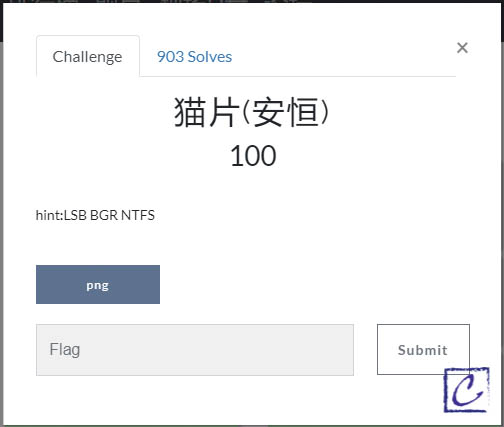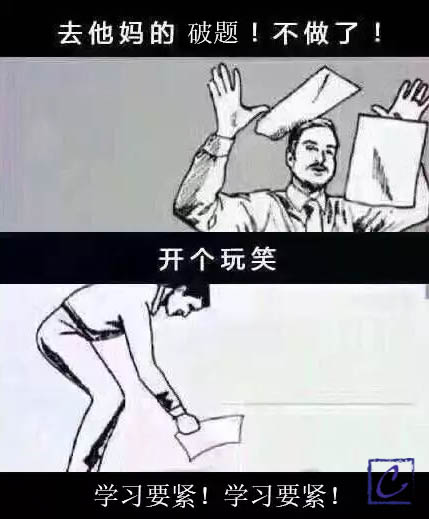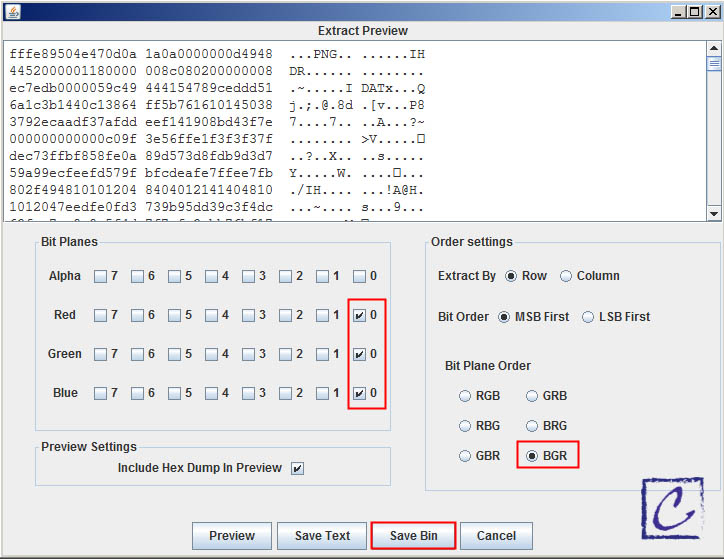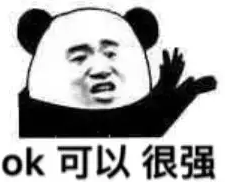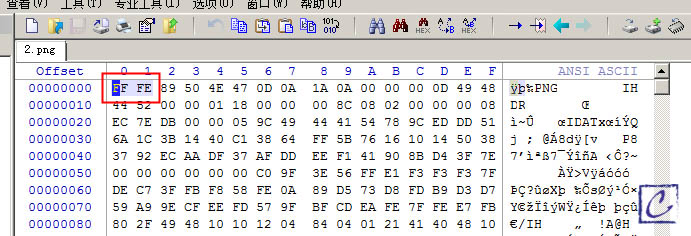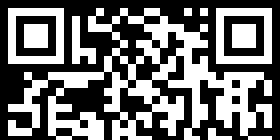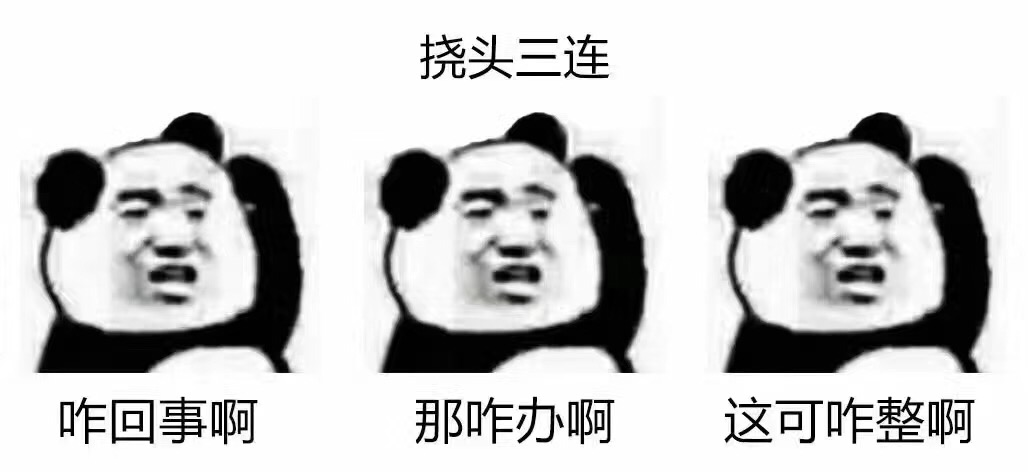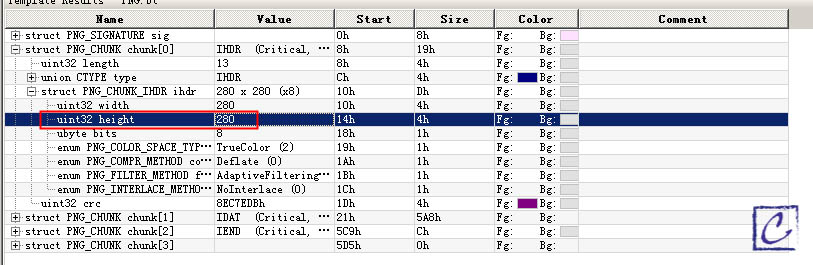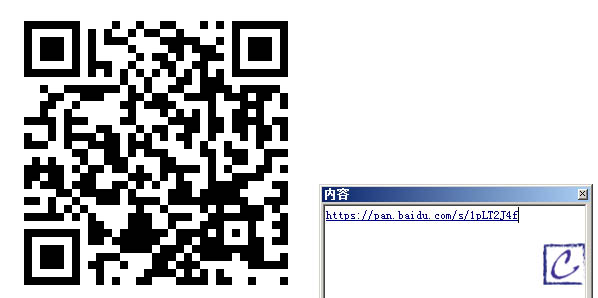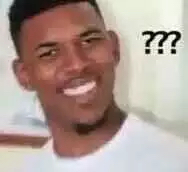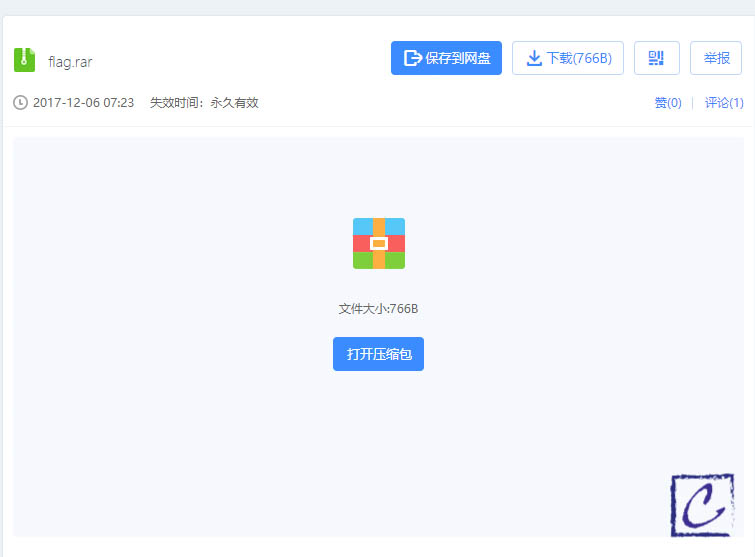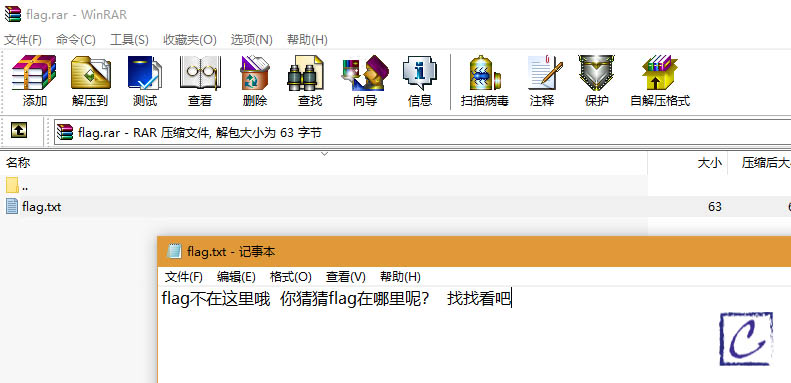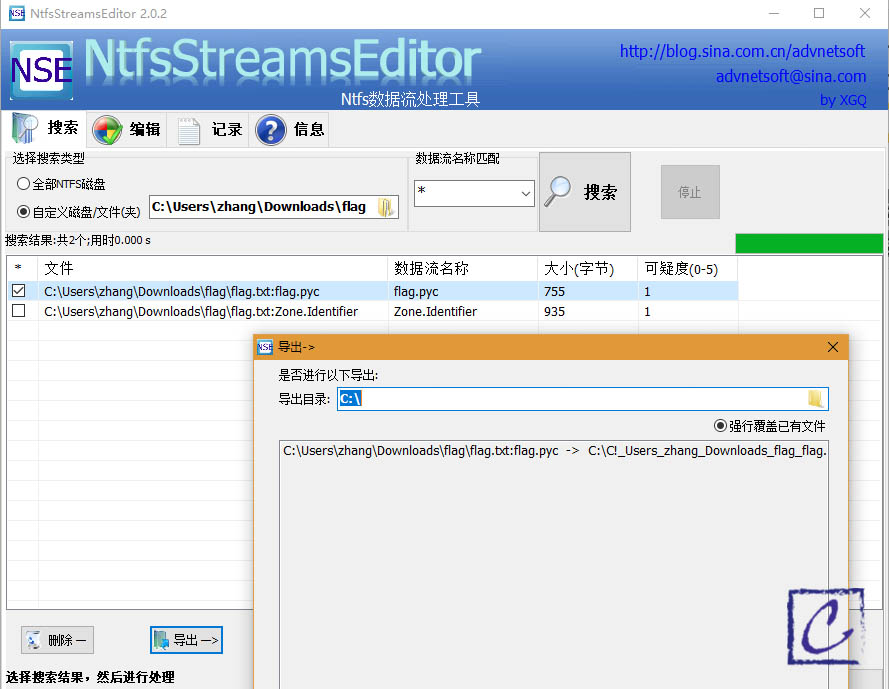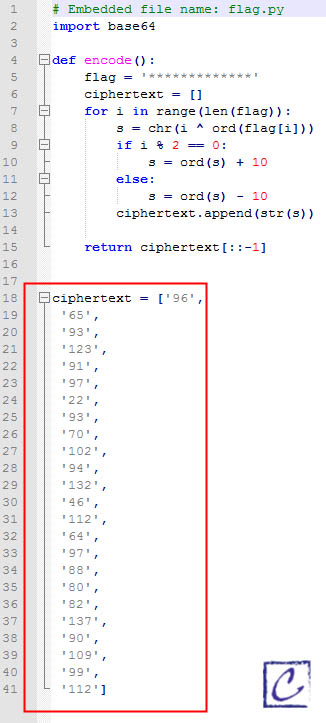encode。。。也就是说我还要写个程序给他decode一下？！多大点事儿嘛，编！（心在痛）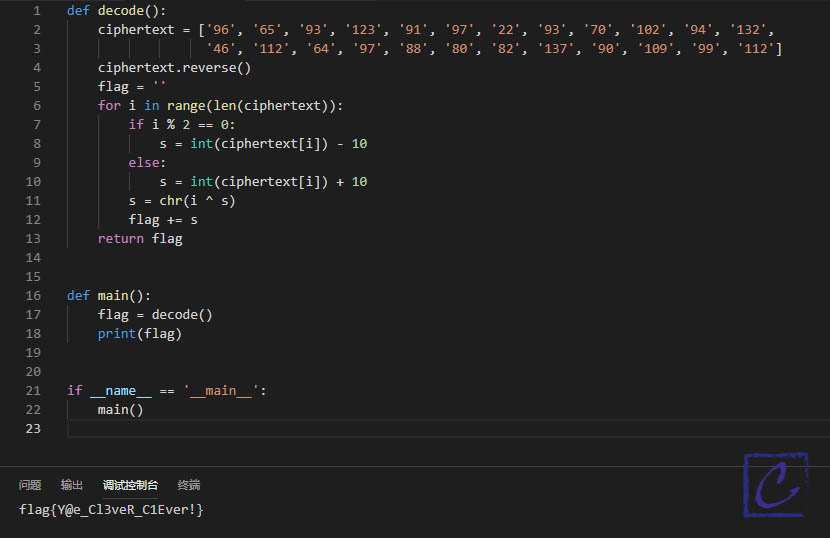``````def decode():
ciphertext = ['96', '65', '93', '123', '91', '97', '22', '93', '70', '102', '94', '132',
'46', '112', '64', '97', '88', '80', '82', '137', '90', '109', '99', '112']
ciphertext.reverse()
flag = ''
for i in range(len(ciphertext)):
if i % 2 == 0:
s = int(ciphertext[i]) - 10
else:
s = int(ciphertext[i]) + 10
s = chr(i ^ s)
flag += s
return flag

def main():
flag = decode()
print(flag)

if __name__ == '__main__':
main()``````（本文为作者原创。转载请注明：转自carlzhang.xyz

*昵称：

*邮箱：

*留言：